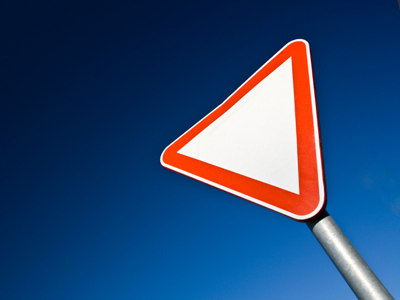The angles of a triangle total 180o.

# Position (Year 6)

In KS2 Year Six, children should be quite comfortable when dealing with position and direction in Maths. By now they should be familiar with degrees and easily able to recognise acute, obtuse and right angles. They should also know that a triangle contains 180 degrees. They will be more familiar with using coordinates on a grid to describe the position of a shape and will now be shown how these change when shapes are rotated or reflected.

Knowing position involves being able to read the coordinates of shapes on a grid. Coordinates are used for map reading and also in knowing where to place a specific point on a grid. But how do the coordinates change when shapes are rotated or reflected? Working out the new coordinates of a rotated shape can be difficult without a pencil and paper!

See how much you remember from your maths classes by trying this quiz all about position.

1.
If I turn 60o how much more do I need to turn to complete one full turn?
100o
200o
300o
400o
360o - 60o = 300o
2.
What is the intersection?
The point where two angles meet
The point where two coordinates meet
The point where two shapes meet
The point where two lines meet
Where two roads meet it is also called an intersection
3.
What do we call two lines that cross one another?
Intersecting
Friends
Meeting
Crisscross
Intersecting lines meet but parallel lines never do
4.
What do the angles of a triangle total?
180o
360o
200o
90o
No matter what lengths its sides are or what shape it is, the angles in a triangle always add up to 180o
5.
A rectangle has the coordinates (2,2) (2,4) (6,4). What is the fourth coordinate?
(2,6)
(6,2)
(1,6)
(4,6)
This rectangle would have its longest sides at the top and bottom
6.
What is used to measure angles?
Ruler
Protractor
Tape measure
Compass
Most protractors are semi-circles divided into 180o
7.
A triangle has the coordinates (1,1) (1,4) (4,1). What will the coordinates be after a quarter turn clockwise?
(1,1) (4,1) (1,-2)
(1,-1) (4,1) (1,-4)
(1,1) (4,1) (1,4)
(1,1) (4,1) (4,-4)
If you worked that out without drawing on some paper then very well done!
8.
If a triangle has a 60o angle and a 40o angle what angle is the third corner?
60o
70o
80o
90o
60o + 40o = 100o so 180o - 100o = 80o
9.
What is the total size of the other two angles in a right angled triangle?
30o
45o
60o
90o
180o - 90o = 90o so the other two angles must add up to that
10.
A shape has the coordinates (5,1) (1,4) (5,6) (9,4). What shape is it?
Square
Rectangle
Rhombus
Kite